Construction of a circle when its radius is known

Chapter 14 Class 6 Practical Geometry
Concept wise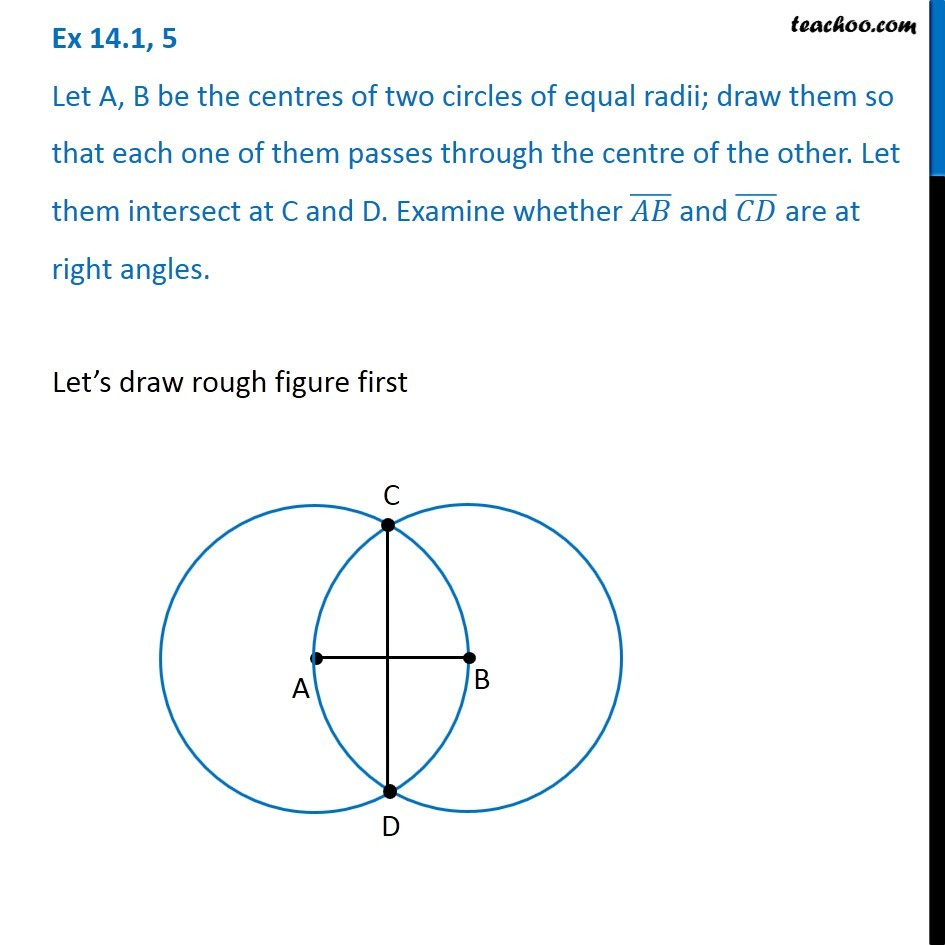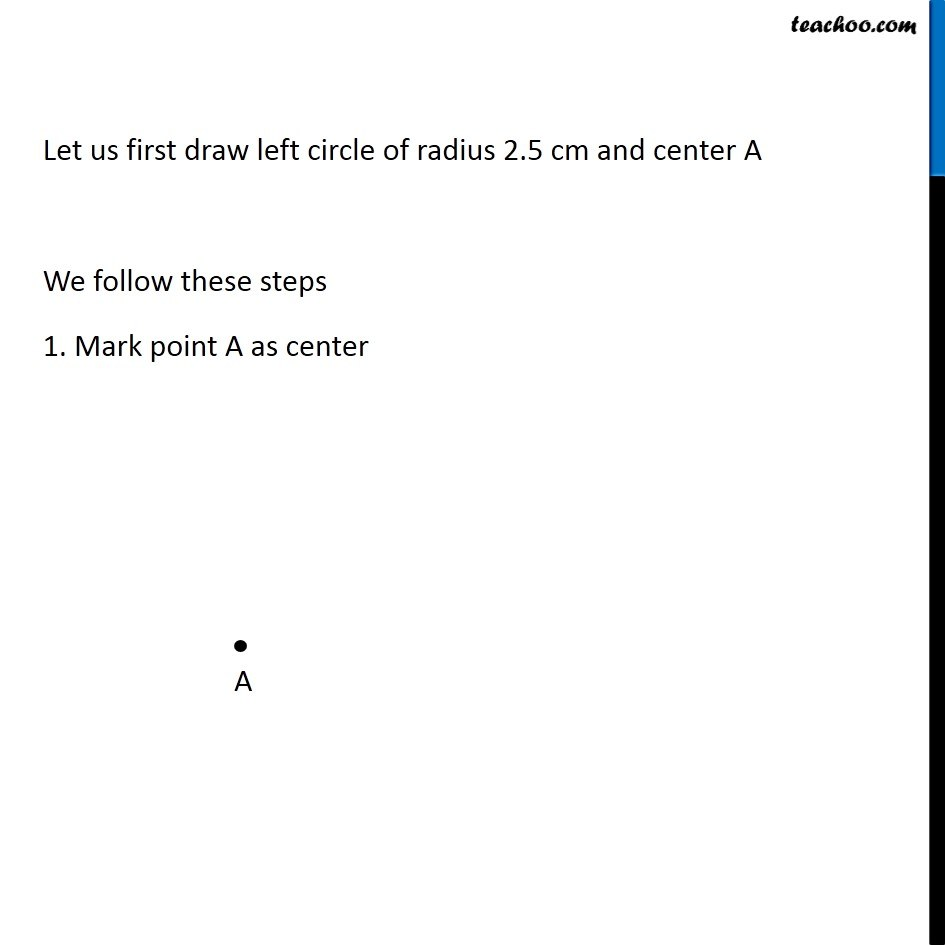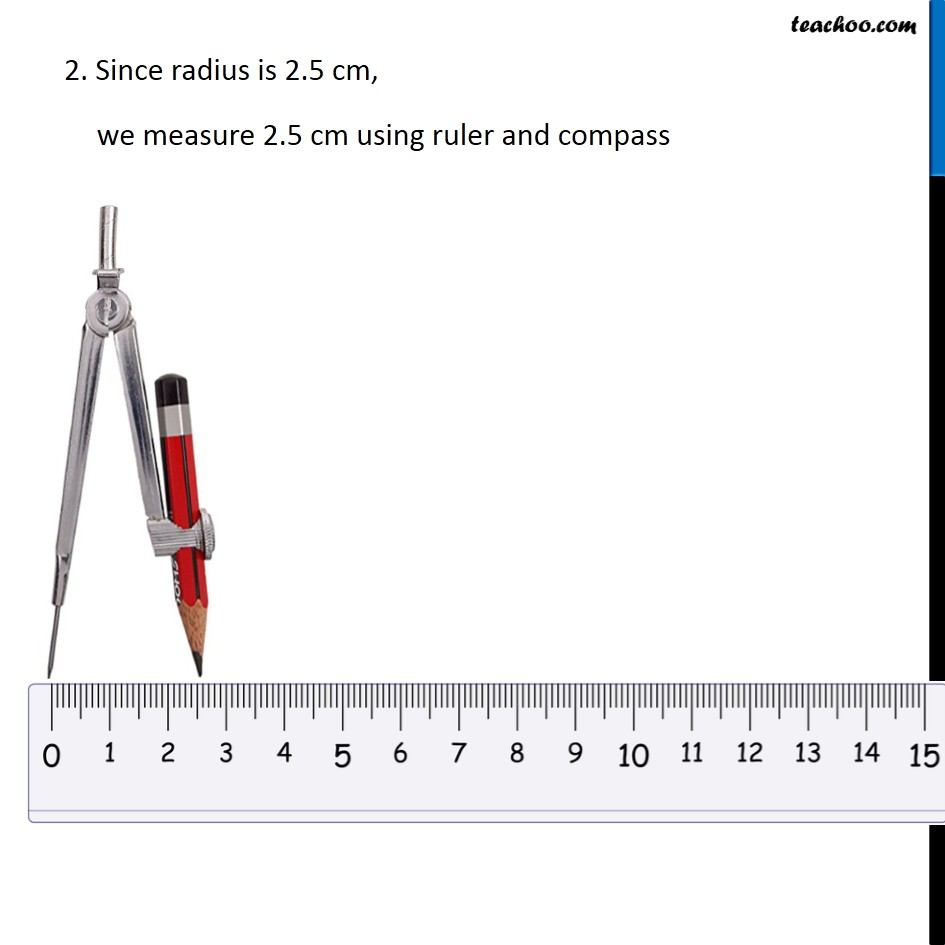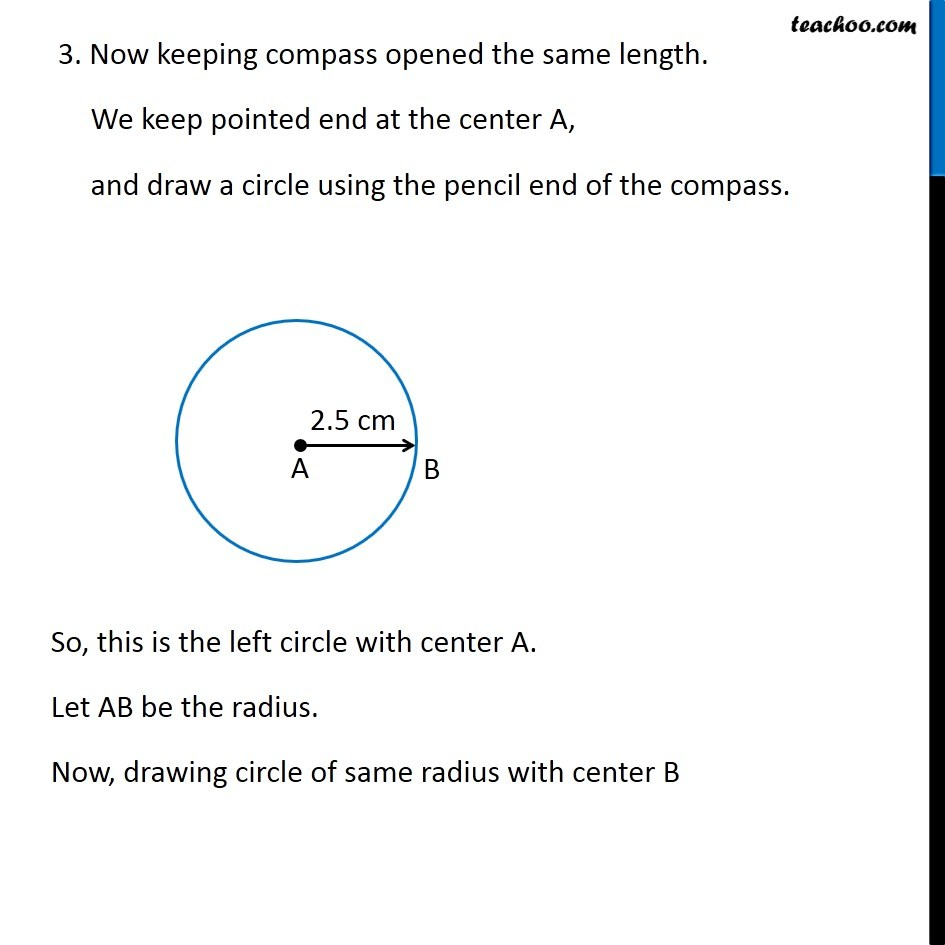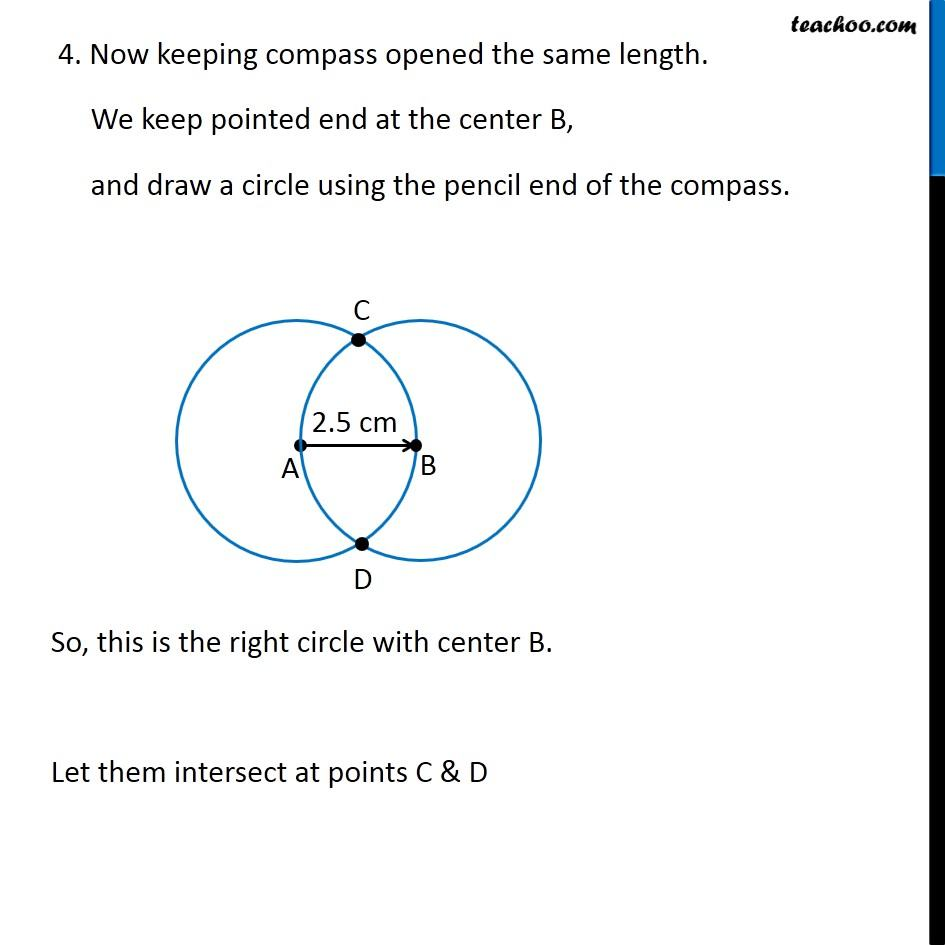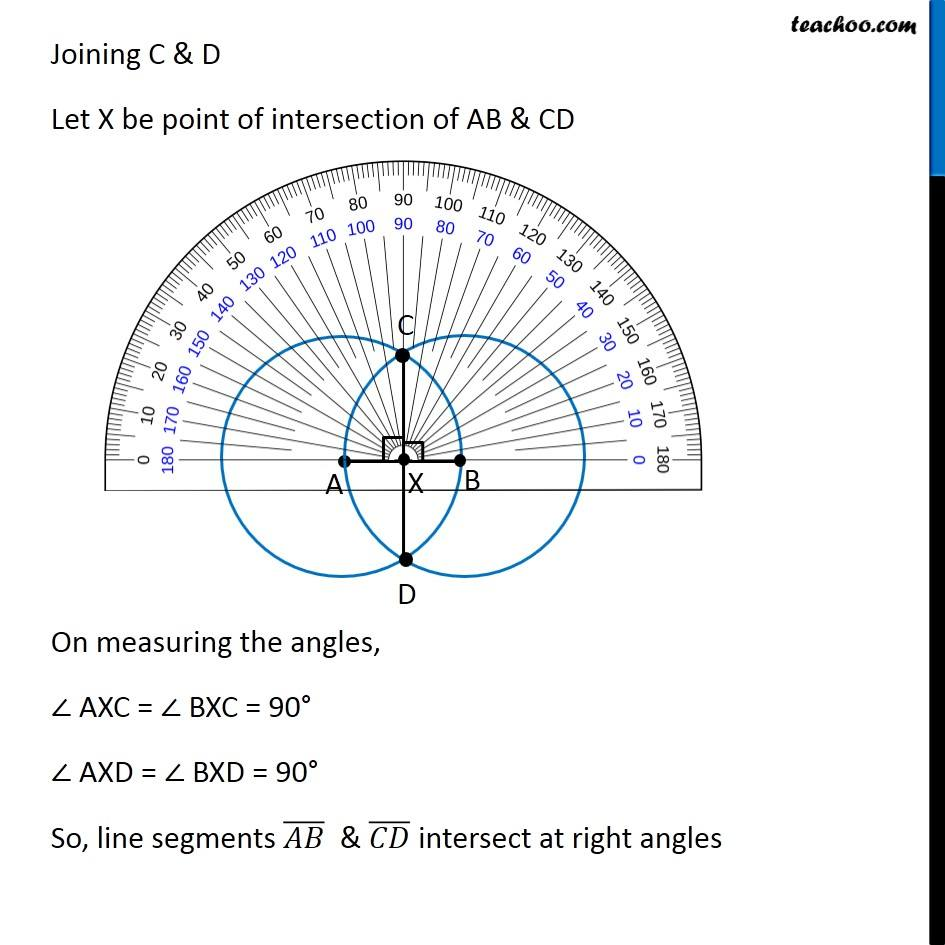### Transcript

Ex 14.1, 5 Let A, B be the centres of two circles of equal radii; draw them so that each one of them passes through the centre of the other. Let them intersect at C and D. Examine whether (𝐴𝐵) ̅" and " (𝐶𝐷) ̅" are at right angles." Let’s draw rough figure first Let us first draw left circle of radius 2.5 cm and center A We follow these steps 1. Mark point A as center 2. Since radius is 2.5 cm, we measure 2.5 cm using ruler and compass 3. Now keeping compass opened the same length. We keep pointed end at the center A, and draw a circle using the pencil end of the compass. So, this is the left circle with center A. Let AB be the radius. Now, drawing circle of same radius with center B 4. Now keeping compass opened the same length. We keep pointed end at the center B, and draw a circle using the pencil end of the compass. So, this is the right circle with center B. Let them intersect at points C & D Joining C & D Let X be point of intersection of AB & CD On measuring the angles, ∠ AXC = ∠ BXC = 90° ∠ AXD = ∠ BXD = 90° So, line segments (𝐴𝐵) ̅ & (𝐶𝐷) ̅ intersect at right angles